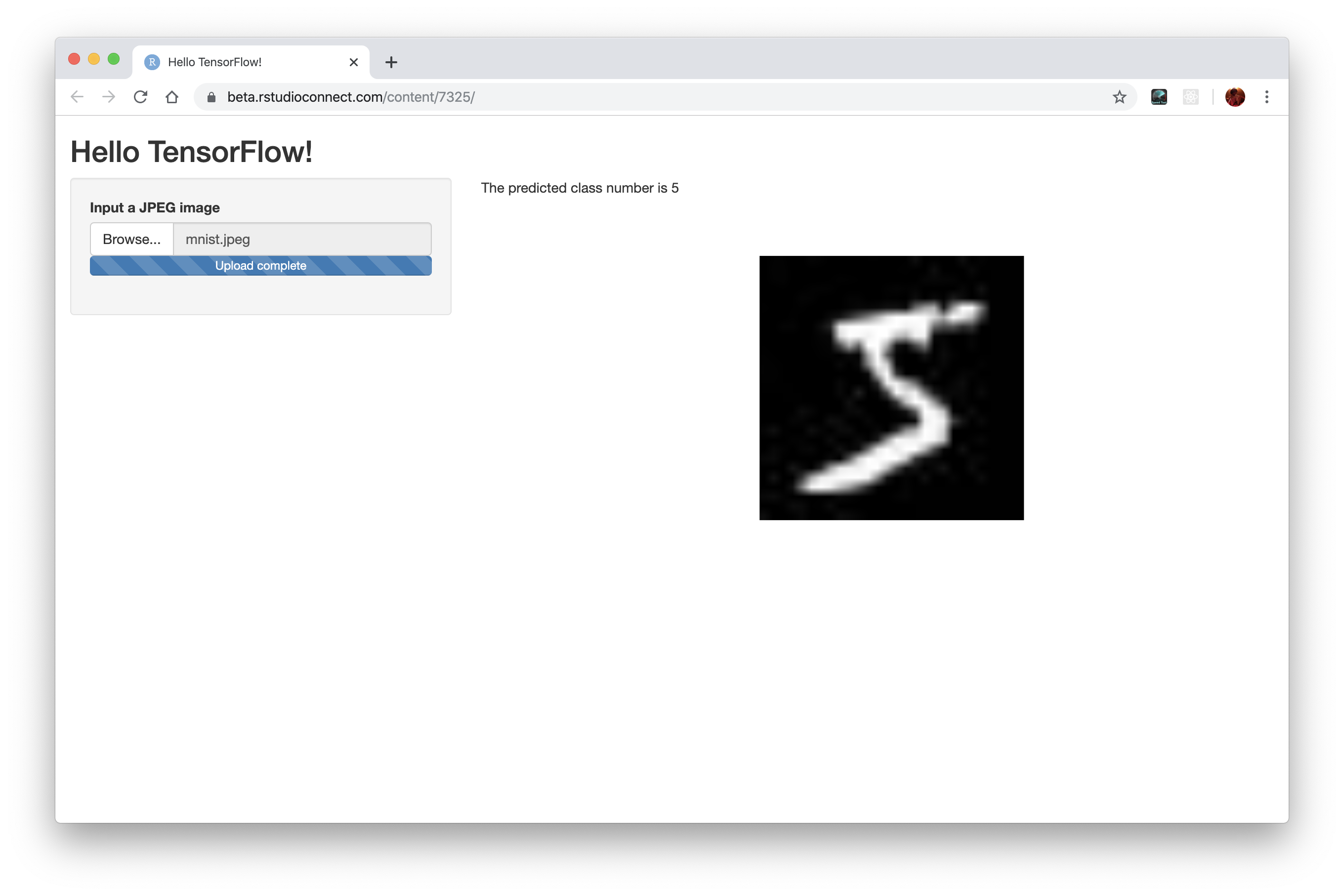# Deploying a Shiny app with a TensorFlow model

In this tutorial you will learn how to deploy a TensorFlow model inside a Shiny app. We will build a model that can classify handwritten digits in images, then we will build a Shiny app that let’s you upload an image and get predictions from this model.

## Building the model

The first thing we are going to do is to build our model. We will use the Keras API to build this model.

We will use the MNIST dataset to build our model.

``````library(keras)
library(tensorflow)
mnist <- dataset_mnist()

mnist\$train\$x <- (mnist\$train\$x/255) %>%
array_reshape(., dim = c(dim(.), 1))

mnist\$test\$x <- (mnist\$test\$x/255) %>%
array_reshape(., dim = c(dim(.), 1))``````

Now, we are going to define our Keras model, it will be a simple convolutional neural network.

``````model <- keras_model_sequential() %>%
layer_conv_2d(filters = 16, kernel_size = c(3,3), activation = "relu") %>%
layer_max_pooling_2d(pool_size = c(2,2)) %>%
layer_conv_2d(filters = 16, kernel_size = c(3,3), activation = "relu") %>%
layer_max_pooling_2d(pool_size = c(2,2)) %>%
layer_flatten() %>%
layer_dense(units = 128, activation = "relu") %>%
layer_dense(units = 10, activation = "softmax")

model %>%
compile(
loss = "sparse_categorical_crossentropy",
metrics = "accuracy"
)``````

Next, we fit the model using the MNIST dataset:

``````model %>%
fit(
x = mnist\$train\$x, y = mnist\$train\$y,
batch_size = 32,
epochs = 5,
validation_sample = 0.2,
verbose = 2
)``````
``````Epoch 1/5
1875/1875 - 7s - loss: 0.1730 - accuracy: 0.9477 - 7s/epoch - 4ms/step
Epoch 2/5
1875/1875 - 5s - loss: 0.0558 - accuracy: 0.9829 - 5s/epoch - 3ms/step
Epoch 3/5
1875/1875 - 4s - loss: 0.0387 - accuracy: 0.9879 - 4s/epoch - 2ms/step
Epoch 4/5
1875/1875 - 4s - loss: 0.0300 - accuracy: 0.9905 - 4s/epoch - 2ms/step
Epoch 5/5
1875/1875 - 5s - loss: 0.0226 - accuracy: 0.9926 - 5s/epoch - 3ms/step``````

When we are happy with our model accuracy in the validation dataset we can `evaluate` the results on the test dataset with:

``model %>% evaluate(x = mnist\$test\$x, y = mnist\$test\$y)``
``313/313 - 0s - loss: 0.0315 - accuracy: 0.9890 - 449ms/epoch - 1ms/step``
``````      loss   accuracy
0.03146039 0.98900002 ``````

OK, we have 99% accuracy on the test dataset and we want to deploy that model. First, let’s save the model in the `SavedModel` format using:

``save_model_tf(model, "cnn-mnist")``

With the model built and saved we can now start building our plumber API file.

## Shiny app

A simple shiny app can be define in an `app.R` file with a few conventions. Here’s how we can structure our Shiny app.

``````library(shiny)
library(keras)

# Define the UI
ui <- fluidPage(
# App title ----
titlePanel("Hello TensorFlow!"),
# Sidebar layout with input and output definitions ----
sidebarLayout(
# Sidebar panel for inputs ----
sidebarPanel(
fileInput("image_path", label = "Input a JPEG image")
),
# Main panel for displaying outputs ----
mainPanel(
# Output: Histogram ----
textOutput(outputId = "prediction"),
plotOutput(outputId = "image")
)
)
)

# Define server logic required to draw a histogram ----
server <- function(input, output) {

image <- reactive({
req(input\$image_path)
})

output\$prediction <- renderText({

img <- image() %>%
array_reshape(., dim = c(1, dim(.), 1))

paste0("The predicted class number is ", predict_classes(model, img))
})

output\$image <- renderPlot({
plot(as.raster(image()))
})

}

shinyApp(ui, server)``````

This app can be used locally or deployed using any Shiny deployment option. If you are deploying to RStudio Connect or Shinnyapps.io, don’t forget to set the `RETICULATE_PYTHON` environment variable so `rsconnect` can detect what python packages are required to reproduce your local environment. See the F.A.Q. for more information.You can see a live version of this app here. Note that to keep the code simple, it will only accept JPEG images with 28x28 pixels. You can download this file if you want to try the app.

When building more advanced models you may not be able to save the entire model using the `save_model_tf` function. In this case you can use the `save_model_weights_tf` function.

For example:

``save_model_weights_tf(model, " cnn-model-weights")``

Then, in the `api.R` file whenn loading the model you will need to rebuild the model using the exact same code that you used when training and saving and then use `load_model_weights_tf` to load the model weights.

``````model <- keras_model_sequential() %>%
layer_conv_2d(filters = 16, kernel_size = c(3,3), activation = "relu") %>%
layer_max_pooling_2d(pool_size = c(2,2)) %>%
layer_conv_2d(filters = 16, kernel_size = c(3,3), activation = "relu") %>%
layer_max_pooling_2d(pool_size = c(2,2)) %>%
layer_flatten() %>%
layer_dense(units = 128, activation = "relu") %>%
layer_dense(units = 10, activation = "softmax")

If you are using Shinyapps.io or RStudio Connect the dependencies will be infered when deploying the app. In this case, don’t forget to set the `RETICULATE_PYTHON` environment variable.